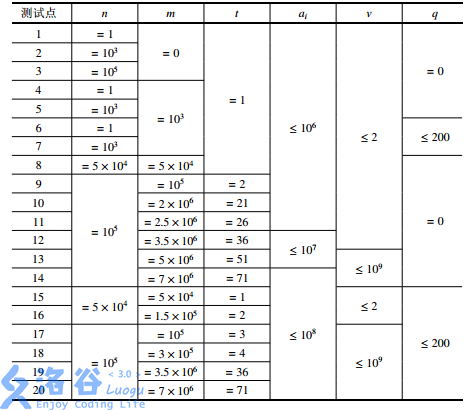# P2827 蚯蚓

• 4.9K通过
• 28.8K提交
• 题目提供者 CCF_NOI
• 评测方式 云端评测
• 标签 二叉堆 NOIp提高组 2016 O2优化 高性能
• 难度 提高+/省选-
• 时空限制 1000ms / 512MB
• 提示：收藏到任务计划后，可在首页查看。

## 题目描述

本题中，我们将用符号 $\lfloor c \rfloor$ 表示对 $c$ 向下取整，例如：$\lfloor 3.0 \rfloor = \lfloor 3.1 \rfloor = \lfloor 3.9 \rfloor = 3$。

蛐蛐国最近蚯蚓成灾了！隔壁跳蚤国的跳蚤也拿蚯蚓们没办法，蛐蛐国王只好去请神刀手来帮他们消灭蚯蚓。

蛐蛐国里现在共有 $n$ 只蚯蚓（$n$ 为正整数）。每只蚯蚓拥有长度，我们设第 $i$ 只蚯蚓的长度为 $a_i$ ($i=1,2,\dots,n$)，并保证所有的长度都是非负整数（即：可能存在长度为 $0$ 的蚯蚓）。

每一秒，神刀手会在所有的蚯蚓中，准确地找到最长的那一只（如有多个则任选一个）将其切成两半。神刀手切开蚯蚓的位置由常数 $p$（是满足 $0 < p < 1$ 的有理数）决定，设这只蚯蚓长度为 $x$，神刀手会将其切成两只长度分别为 $\lfloor px \rfloor$ 和 $x - \lfloor px \rfloor$ 的蚯蚓。特殊地，如果这两个数的其中一个等于 $0$，则这个长度为 $0$ 的蚯蚓也会被保留。此外，除了刚刚产生的两只新蚯蚓，其余蚯蚓的长度都会增加 $q$（是一个非负整常数）。

蛐蛐国王知道这样不是长久之计，因为蚯蚓不仅会越来越多，还会越来越长。蛐蛐国王决定求助于一位有着洪荒之力的神秘人物，但是救兵还需要 $m$ 秒才能到来……（$m$ 为非负整数）

蛐蛐国王希望知道这 $m$ 秒内的战况。具体来说，他希望知道：

• $m$ 秒内，每一秒被切断的蚯蚓被切断前的长度（有 $m$ 个数）；
• $m$ 秒后，所有蚯蚓的长度（有 $n + m$ 个数）。

蛐蛐国王当然知道怎么做啦！但是他想考考你……

## 输入输出格式

输入格式：

第一行包含六个整数 $n,m,q,u,v,t$，其中：$n,m,q$ 的意义见【问题描述】；$u,v,t$ 均为正整数；你需要自己计算 $p=u / v$（保证 $0 < u < v$）；$t$ 是输出参数，其含义将会在【输出格式】中解释。

第二行包含 $n$ 个非负整数，为 $a_1, a_2, \dots, a_n$，即初始时 $n$ 只蚯蚓的长度。

同一行中相邻的两个数之间，恰好用一个空格隔开。

保证 $1 \leq n \leq 10^5$，$0 \leq m \leq 7 \times 10^6$，$0 < u < v \leq 10^9$，$0 \leq q \leq 200$，$1 \leq t \leq 71$，$0 \leq a_i \leq 10^8$。

输出格式：

第一行输出 $\left \lfloor \frac{m}{t} \right \rfloor$ 个整数，按时间顺序，依次输出第 $t$ 秒，第 $2t$ 秒，第 $3t$ 秒，……被切断蚯蚓（在被切断前）的长度。

第二行输出 $\left \lfloor \frac{n+m}{t} \right \rfloor$ 个整数，输出 $m$ 秒后蚯蚓的长度；需要按从大到小的顺序，依次输出排名第 $t$，第 $2t$，第 $3t$，……的长度。

同一行中相邻的两个数之间，恰好用一个空格隔开。即使某一行没有任何数需要输出，你也应输出一个空行。

请阅读样例来更好地理解这个格式。

## 输入输出样例

输入样例#1： 复制
3 7 1 1 3 1
3 3 2
输出样例#1： 复制
3 4 4 4 5 5 6
6 6 6 5 5 4 4 3 2 2
输入样例#2： 复制
3 7 1 1 3 2
3 3 2
输出样例#2： 复制
4 4 5
6 5 4 3 2
输入样例#3： 复制
3 7 1 1 3 9
3 3 2
输出样例#3： 复制
//空行
2

## 说明

【样例解释1】

在神刀手到来前：$3$只蚯蚓的长度为$3,3,2$。

$1$秒后：一只长度为$3$的蚯蚓被切成了两只长度分别为$1$和$2$的蚯蚓，其余蚯蚓的长度增加了$1$。最终$4$只蚯蚓的长度分别为$(1,2),4,3$。括号表示这个位置刚刚有一只蚯蚓被切断

$2$秒后：一只长度为$4$的蚯蚓被切成了$1$和$3$。$5$只蚯蚓的长度分别为：$2,3,(1,3),4$。

3秒后：一只长度为$4$的蚯蚓被切断。$6$只蚯蚓的长度分别为：$3,4,2,4,(1,3)$。

$4$秒后：一只长度为$4$的蚯蚓被切断。$7$只蚯蚓的长度分别为：$4,(1,3),3,5,2,4$。

$5$秒后：一只长度为$5$的蚯蚓被切断。$8$只蚯蚓的长度分别为：$5,2,4,4,(1,4),3,5$。

$6$秒后：一只长度为$5$的蚯蚓被切断。$9$只蚯蚓的长度分别为：$(1,4),3,5,5,2,5,4,6$。

$7$秒后：一只长度为$6$的蚯蚓被切断。$10$只蚯蚓的长度分别为：$2,5,4,6,6,3,6,5,(2,4)$。所以，$7$秒内被切断的蚯蚓的长度依次为$3,4,4,4,5,5,6$。$7$秒后，所有蚯蚓长度从大到小排序为$6,6,6,5,5,4,4,3,2,2$

【样例解释2】

这个数据中只有$t=2$与上个数据不同。只需在每行都改为每两个数输出一个数即可。

虽然第一行最后有一个$6$没有被输出，但是第二行仍然要重新从第二个数再开始输出。

【样例解释3】

这个数据中只有$t=9$与上个数据不同。

注意第一行没有数要输出，但也要输出一个空行。

【数据范围】提示
标程仅供做题后或实在无思路时参考。
请自觉、自律地使用该功能并请对自己的学习负责。
如果发现恶意抄袭标程，将按照I类违反进行处理。